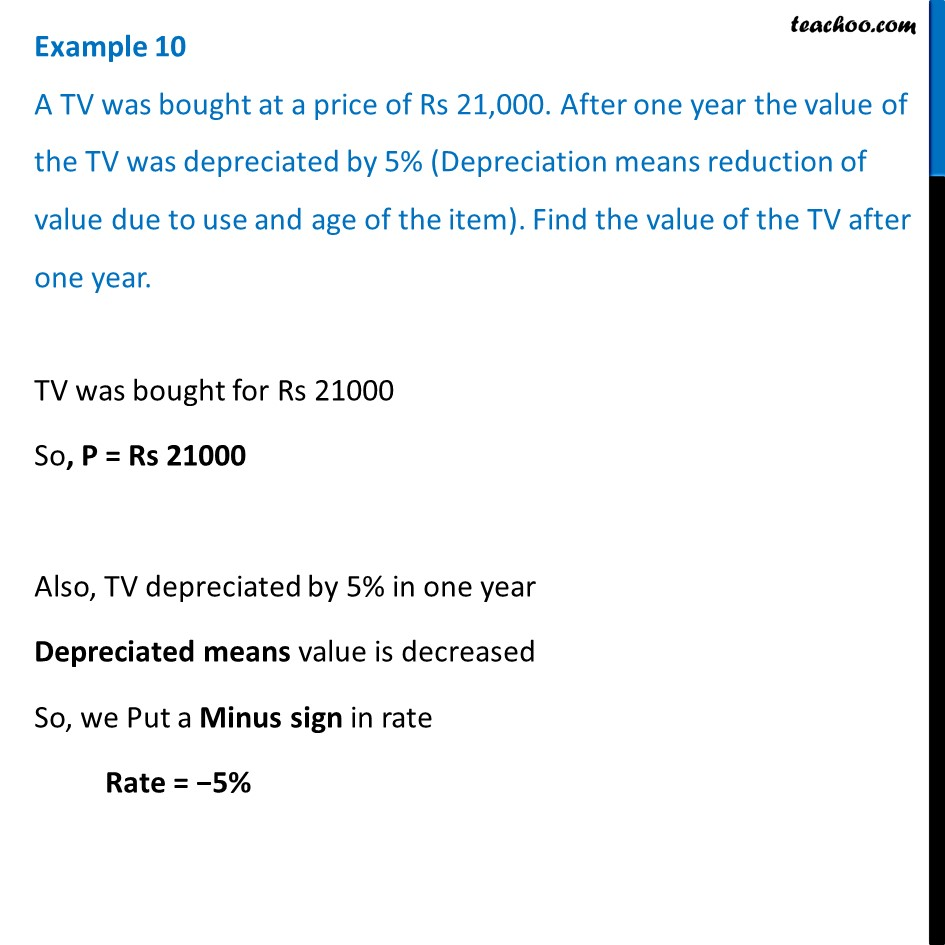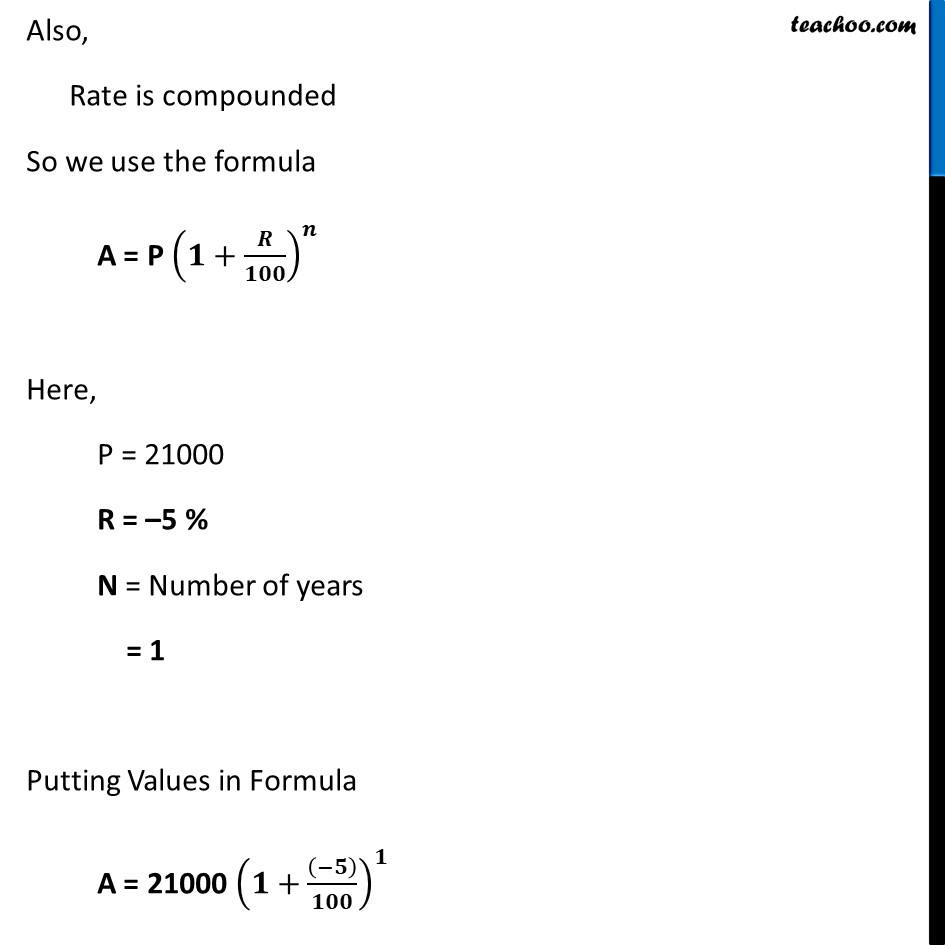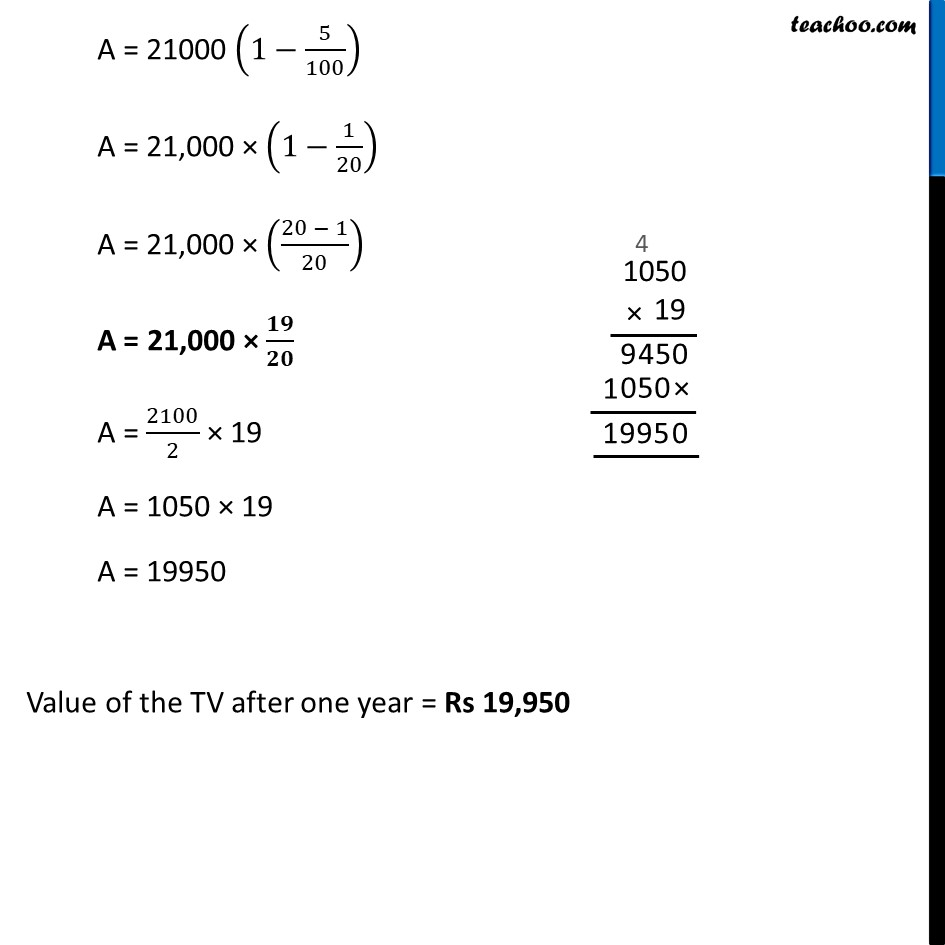Examples

Chapter 7 Class 8 Comparing Quantities
Serial order wiseLearn in your speed, with individual attention - Teachoo Maths 1-on-1 Class

### Transcript

Example 10 A TV was bought at a price of Rs 21,000. After one year the value of the TV was depreciated by 5% (Depreciation means reduction of value due to use and age of the item). Find the value of the TV after one year.TV was bought for Rs 21000 So, P = Rs 21000 Also, TV depreciated by 5% in one year Depreciated means value is decreased So, we Put a Minus sign in rate Rate = −5% Also, Rate is compounded So we use the formula A = P (𝟏+𝑹/𝟏𝟎𝟎)^𝒏 Here, P = 21000 R = –5 % N = Number of years = 1 Putting Values in Formula A = 21000 (𝟏+((−𝟓))/𝟏𝟎𝟎)^𝟏 A = 21000 (1−5/100) A = 21,000 × (1−1/20) A = 21,000 × ((20 − 1)/20) A = 21,000 × 𝟏𝟗/𝟐𝟎 A = 2100/2 × 19 A = 1050 × 19 A = 19950 Value of the TV after one year = Rs 19,950